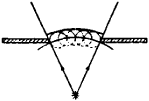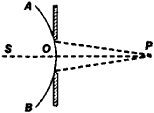Huygens-Fresnel Principle

Also found in: Wikipedia.

Huygens-Fresnel principle

[¦hī·gənz frā′nel ‚prin·sə·pəl]
(optics)
A modification of Huygens' principle according to which the amplitude of secondary waves falls off in proportion to the cosine of the angle between the normals to the original and secondary waves, and the secondary waves interfere with each other according to the principle of superposition. Also known as Fresnel-Huygens principle.
McGraw-Hill Dictionary of Scientific & Technical Terms, 6E, Copyright © 2003 by The McGraw-Hill Companies, Inc.
The following article is from The Great Soviet Encyclopedia (1979). It might be outdated or ideologically biased.

Huygens-Fresnel Principle

an approximate method for solving problems of propagation of waves, particularly light waves. According to C. Huygens’ initial principle (1678), each element of a surface that a wave reaches at a particular moment is the center of elementary waves whose envelope forms the wave surface at the next instant (Figure 1). The backward elementary waves (dotted line in Figure 1) must be disregarded. This principle makes it possible to simplify the problem of determining at any point the effect of an entire wave process occurring at some volume of space, reducing it to the calculation of the action of an arbitrarily chosen wave surface at the given point. Huygens’ principle explains the propagation of waves, agreeing with the laws of geometrical optics, but it does not explain the phenomena of diffraction. In 1815, A. J. Fresnel completed Huygens’ principle by introducing the notion of the coherence of elementary waves and their interference.Figure 1Figure 2

According to the Huygens-Fresnel principle, a wave disturbance at some point P(Figure 2) may be regarded as the result of the interference of elementary secondary waves that are radiated by each element of some wave surface. In Figure 2 such a surface is the spherical surface of the wave AOB radiated by point source S. If the propagation of waves that is bounded by some obstacle (for example, a hole in an opaque screen as in Figure 2) is considered, it is appropriate to choose a wave surface such that it touches the edges of the obstacle.

The Huygens-Fresnel principle gives an approximate treatment of many cases of diffraction.

REFERENCE

Landsberg, G. S. Optika, 4th ed. Moscow-Leningrad, 1957. (Ob-shchii kurs fiziki, vol. 3.)